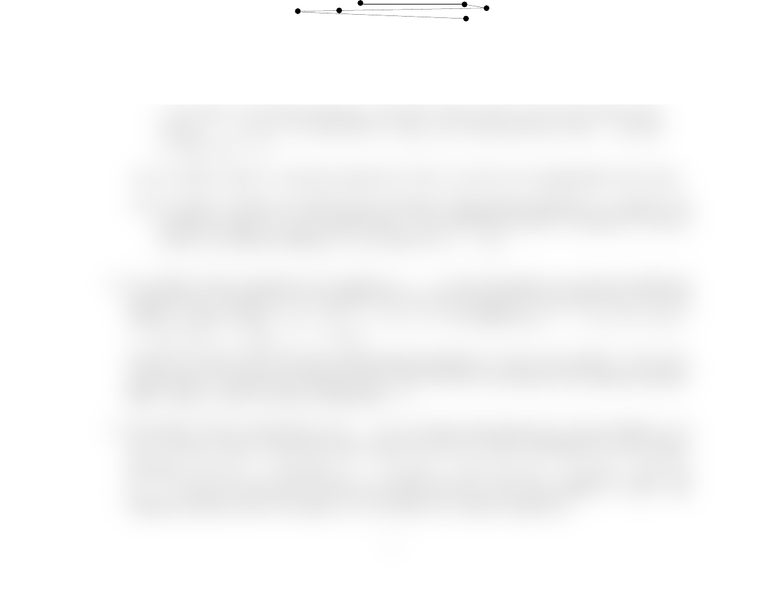# Assignment #4 This is the forth assignment from winter 2010 term

32 views2 pages
School
University of Waterloo
Department
Computer Science
Course
CS341CS 341, Winter 2010
Timothy Chan
Assignment 4 (due March 23 Tuesday noon)
instructions and http://www.student.cs.uwaterloo.ca/~cs341/prog.html for programming
guidelines.
1. [17 marks] Given a “chain” with nlinks of lengths a1,...,an, where each aiis a positive
integer, we want to “fold” the chain (in one dimension) so that the length of the folded chain
is minimized. More formally, we want to minimize Wsubject to the following constraint:
there exist tin the interval [0, W ] and s1,...,sn∈ {−1,+1}such that t+Pj
i=1 siai[0, W ]
for all j∈ {0,...,n}. (Here, tdenotes the position of the ﬁrst joint, and si=±1 depending
on whether we turn rightward or leftward for the i-th link.)
Example: for a1= 5, a2= 1, a3= 7, a4= 2, a5= 8, the minimum length Wis 9.
8
27
1
5
Deﬁne the following subproblem, given 0 kn, 0 `m:
Let A[k, `, m] be true if we can fold the chain with klinks of lengths a1, . . . , akso
that all the joints lie in the interval [0, m] and the position of the last joint is exactly
`. Let A[k, `, m] be false otherwise. (In other words, A[k, `, m] is true iﬀ there exist
tand s1,...,sk∈ {−1,1}such that t+Pj
i=1 siai[0, m] for all j∈ {0, . . . , k}and
t+Pk
i=1 siai=`.)
(a) [8 marks] Derive a recursive formula for A[k, `, m] and set up appropriate base cases.
(b) [9 marks] Present an O(nW 2)-time dynamic programming algorithm to compute the
minimum length Wof the folded chain. Give pseudocode both to compute Wand to
retrieve an optimal folding (i.e., the values of s1,...,sn).
2. [15 marks] Given a sequence of nnumbers a1,...,anand an integer k, we want to divide the
sequence into kblocks so as to minimize the sum of the squares of the block sums. In other
words, we want to ﬁnd 1 < i1< i2<···< ik1< n to minimize (a1+···+ai11)2+ (ai1+
···+ai21)2+···+ (aik1+···+an)2.
Present an O(kn2)-time dynamic programming algorithm to solve the problem. Give pseu-
docode both to compute the minimum value and to retrieve the blocks in the optimal solution.
[Hint: deﬁne a class of O(nk) subproblems. . . ]
3. [10 marks] Given nintervals [a1, b1],...,[an, bn] where each interval [ai, bi] has weight wi, we
want to ﬁnd a subset of intervals whose union covers [0,1] while minimizing the total weight.
Example: if [a1, b1] = [0.2,0.6], w1= 1, [a2, b2] = [0.1,1.1], w2= 3, [a3, b3] = [0.5,1.2],
w3= 1, then we would pick the ﬁrst and third intervals, with total weight 2. [Note: this
weighted problem does not appear to be solvable by a greedy approach.]
1
Unlock document

This preview shows half of the first page of the document.
Unlock all 2 pages and 3 million more documents.